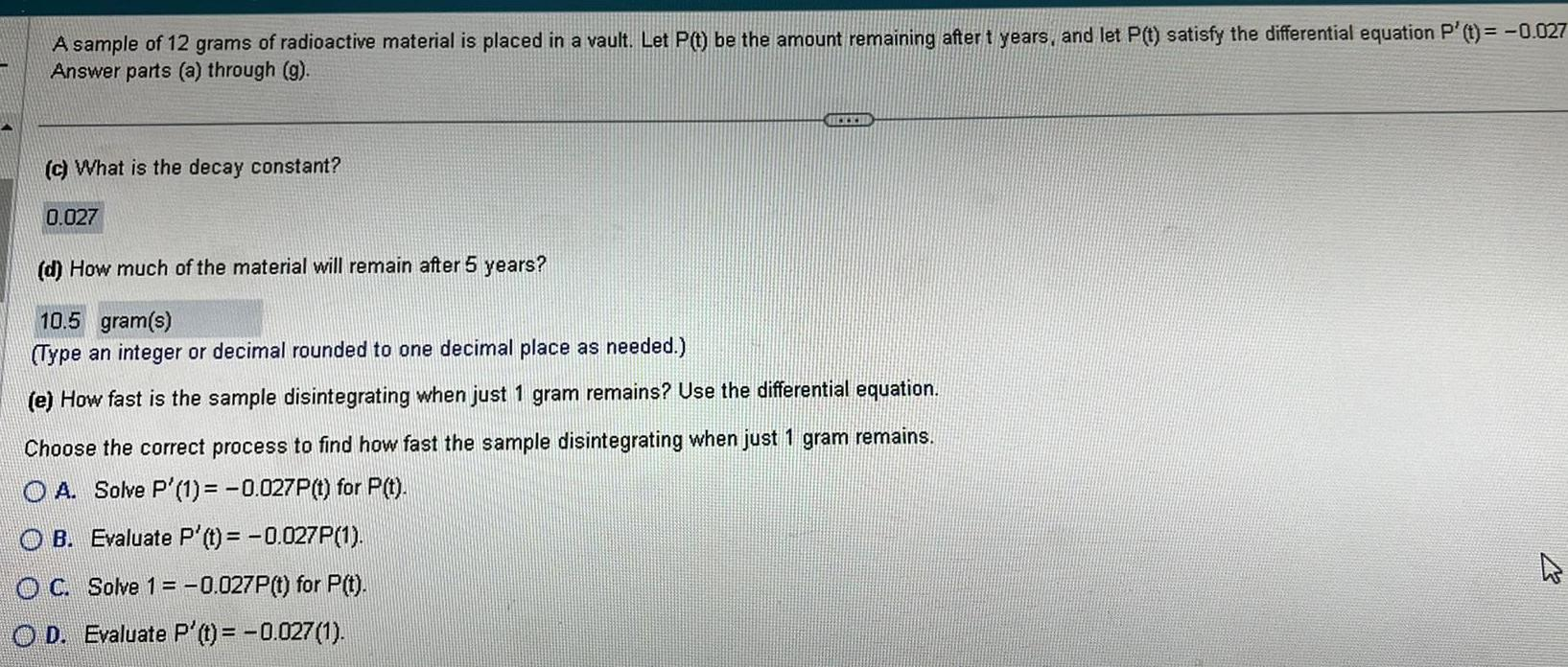Question:

# A sample of 12 grams of radioactive material is placed in a

Last updated: 9/18/2023A sample of 12 grams of radioactive material is placed in a vault Let P t be the amount remaining after t years and let P t satisfy the differential equation P t 0 027 Answer parts a through g c What is the decay constant 0 027 d How much of the material will remain after 5 years 10 5 gram s Type an integer or decimal rounded to one decimal place as needed e How fast is the sample disintegrating when just 1 gram remains Use the differential equation Choose the correct process to find how fast the samp disintegrating when just 1 gram remains OA Solve P 1 0 027P t for P t OB Evaluate P t 0 027P 1 OC Solve 1 0 027P t for P t OD Evaluate P t 0 027 1 D